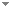Switch to: References

# The Fan Theorem and Unique Existence of Maxima

Journal of Symbolic Logic 71 (2):713 - 720 (2006)

 Unique Solutions.Peter Schuster - 2006 - Mathematical Logic Quarterly 52 (6):534-539.It is folklore that if a continuous function on a complete metric space has approximate roots and in a uniform manner at most one root, then it actually has a root, which of course is uniquely determined. Also in Bishop's constructive mathematics with countable choice, the general setting of the present note, there is a simple method to validate this heuristic principle. The unique solution even becomes a continuous function in the parameters by a mild modification of the uniqueness hypothesis. (...) Direct download     Export citationBookmark6 citations Constructing Local Optima on a Compact Interval.Douglas S. Bridges - 2007 - Archive for Mathematical Logic 46 (2):149-154.The existence of either a maximum or a minimum for a uniformly continuous mapping f of a compact interval into ${\mathbb{R}}$ is established constructively under the hypotheses that f′ is sequentially continuous and f has at most one critical point. Direct download (3 more)     Export citationBookmark1 citation Toward a Clarity of the Extreme Value Theorem.Karin U. Katz, Mikhail G. Katz & Taras Kudryk - 2014 - Logica Universalis 8 (2):193-214.We apply a framework developed by C. S. Peirce to analyze the concept of clarity, so as to examine a pair of rival mathematical approaches to a typical result in analysis. Namely, we compare an intuitionist and an infinitesimal approaches to the extreme value theorem. We argue that a given pre-mathematical phenomenon may have several aspects that are not necessarily captured by a single formalisation, pointing to a complementarity rather than a rivalry of the approaches. Direct download (6 more)     Export citationBookmark2 citations Convexity and Unique Minimum Points.Josef Berger & Gregor Svindland - 2019 - Archive for Mathematical Logic 58 (1-2):27-34.We show constructively that every quasi-convex, uniformly continuous function \ with at most one minimum point has a minimum point, where C is a convex compact subset of a finite dimensional normed space. Applications include a result on strictly quasi-convex functions, a supporting hyperplane theorem, and a short proof of the constructive fundamental theorem of approximation theory. No categories Direct download (2 more)     Export citationBookmark1 citation